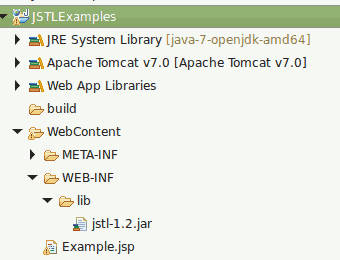# JSTL Less Than Equal(<=), Greater Than Equal(>=)

JSTL Less Than Equal(<=), Greater Than Equal(>=) explains about how to use logical conditions(less than, greater equal etc) with JSTL tag

Consider a JSP Page where you need to check a value whether it is less than, greater than etc and process accordingly, in that scenario,  you can follow this example.

On the following table, I am showing 2 different ways (Method 1 & Method 2), you can achieve logical conditions in JSTL

Logical operation JSTL Method 1 JSTL Method 2
less than lt <
greater than gt >
less than or equal le <=
greater than or equals ge >=
###### Required Libraries

Following jar must be in classpath

1. jstl-1.2.jar

## Project Structure## JSTL Less Than Equal(le), Greater Than Equal(ge) (Method 1)

```<%@ taglib uri="http://java.sun.com/jsp/jstl/core" prefix="c"%>
<%@ taglib uri="http://java.sun.com/jsp/jstl/functions" prefix="fn"%>
<html>
<title>Check Less Than equal(le), Greater Than equal(ge)</title>
<body>

<%
// you can also set the values into request scope same as using c:set
request.setAttribute("count", 5);
%>
<!-- you can check "less than(<)"  -->
<c:if test="\${count lt 6}">
count is less than 6<br />
</c:if>

<!-- you can check "greater than(>)"  -->
<c:if test="\${count gt 4}">
count is greater than 4<br />
</c:if>

<!-- you can check "less than or equals(<=)"  -->
<c:if test="\${count le 5}">
count is less than or equal to 5.<br />
</c:if>

<!-- you can check "greater than or equals(>=)" -->
<c:if test="\${count ge 5}">
count is greater than or equal to 5.<br />
</c:if>

</body>
</html>
```
###### Output
count is less than 6
count is greater than 4
count is less than or equal to 5
count is greater than or equal to 5

## JSTL Less Than Equal(le), Greater Than Equal(ge) (Method 2)

```<%@ taglib uri="http://java.sun.com/jsp/jstl/core" prefix="c"%>
<%@ taglib uri="http://java.sun.com/jsp/jstl/functions" prefix="fn"%>
<html>
<title>Check Less Than equal(<=), Greater Than equal(>=)</title>
<body>

<%
// you can also set the values into request scope same as using c:set
request.setAttribute("count", 5);
%>
<!-- you can check "less than(<)"  -->
<c:if test="\${count < 6}">
count is less than 6<br />
</c:if>

<!-- you can check "greater than(>)"  -->
<c:if test="\${count > 4}">
count is greater than 4<br />
</c:if>

<!-- you can check "less than or equals(<=)"  -->
<c:if test="\${count <= 5}">
count is less than or equal to 5.<br />
</c:if>

<!-- you can check "greater than or equals(>=)" -->
<c:if test="\${count >= 5}">
count is greater than or equal to 5.<br />
</c:if>

</body>
</html>
```
###### Output
count is less than 6
count is greater than 4
count is less than or equal to 5
count is greater than or equal to 5